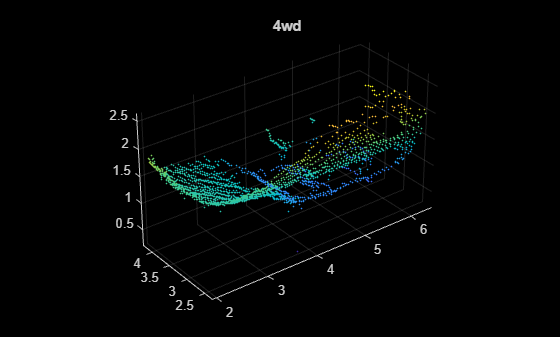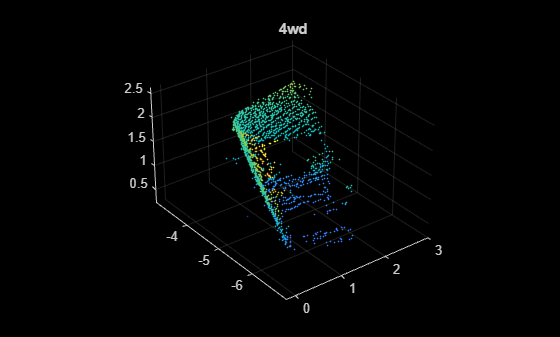# Augment Point Cloud Data For Deep Learning

This example demonstrates how to setup a basic randomized data augmentation pipeline when working with point cloud data in deep learning based workflows. Data augmentation is almost always desirable when working with deep learning because it helps to reduce overfitting during training and can add robustness to types of data transformations which may not be well represented in the original training data.

### Import point cloud data

```dataPath = downloadSydneyUrbanObjects(tempdir); dsTrain = sydneyUrbanObjectsClassificationDatastore(dataPath); dataOut = preview(dsTrain)```
```dataOut=1×2 cell array {1×1 pointCloud} {[4wd]} ```

The datastore `dsTrain` yields a `pointCloud` object and an associated scalar categorical label for each observation.

```figure pcshow(dataOut{1}); title(dataOut{2});```### Define augmentation pipeline

The transform function of a datastore is a convenient tool for defining augmentation pipelines.

`dsAugmented = transform(dsTrain,@augmentPointCloud);`

The `augmentPointCloud `function shown below, applies randomized rotation, homogenous scale, randomized reflection across the x- and y-axes, and randomized per point jitter to each observation using the `randomAffine3d` function to construct randomized affine transformations and the `pctransform` function to apply these transformations to each input point cloud.

`dataOut = preview(dsAugmented)`
```dataOut=1×2 cell array {1×1 pointCloud} {[4wd]} ```

It is always a good idea to visually inspect the data that comes out of any augmentation that is done on training data to make sure that the data looks as expected. The point cloud below is the same as the original shown previously, but with randomized affine warping with per point jitter added.

```figure pcshow(dataOut{1}); title(dataOut{2});```The resulting `TransformedDatastore` and `dsAugmented` can be passed to deep learning functions including `trainNetwork`, `predict`, and `classify` for use in training and inference.

### Supporting Functions

```function datasetPath = downloadSydneyUrbanObjects(dataLoc) if nargin == 0 dataLoc = pwd(); end dataLoc = string(dataLoc); url = "http://www.acfr.usyd.edu.au/papers/data/"; name = "sydney-urban-objects-dataset.tar.gz"; if ~exist(fullfile(dataLoc,'sydney-urban-objects-dataset'),'dir') disp('Downloading Sydney Urban Objects Dataset...'); untar(fullfile(url,name),dataLoc); end datasetPath = dataLoc.append('sydney-urban-objects-dataset'); end function ds = sydneyUrbanObjectsClassificationDatastore(datapath,folds) % sydneyUrbanObjectsClassificationDatastore Datastore with point clouds and % associated categorical labels for Sydney Urban Objects dataset. % % ds = sydneyUrbanObjectsDatastore(datapath) constructs a datastore that % represents point clouds and associated categories for the Sydney Urban % Objects dataset. The input, datapath, is a string or char array which % represents the path to the root directory of the Sydney Urban Objects % Dataset. % % ds = sydneyUrbanObjectsDatastore(___,folds) optionally allows % specification of desired folds that you wish to be included in the % output ds. For example, [1 2 4] specifies that you want the first, % second, and fourth folds of the Dataset. Default: [1 2 3 4]. if nargin < 2 folds = 1:4; end datapath = string(datapath); path = fullfile(datapath,'objects',filesep); % For now, include all folds in Datastore foldNames{1} = importdata(fullfile(datapath,'folds','fold0.txt')); foldNames{2} = importdata(fullfile(datapath,'folds','fold1.txt')); foldNames{3} = importdata(fullfile(datapath,'folds','fold2.txt')); foldNames{4} = importdata(fullfile(datapath,'folds','fold3.txt')); names = foldNames(folds); names = vertcat(names{:}); fullFilenames = append(path,names); ds = fileDatastore(fullFilenames,'ReadFcn',@extractTrainingData,'FileExtensions','.bin'); end function dataOut = extractTrainingData(fname) [pointData,intensity] = readbin(fname); [~,name] = fileparts(fname); name = string(name); name = extractBefore(name,'.'); labelNames = ["4wd","bench","bicycle","biker",... "building","bus","car","cyclist","excavator","pedestrian","pillar",... "pole","post","scooter","ticket_machine","traffic_lights","traffic_sign",... "trailer","trash","tree","truck","trunk","umbrella","ute","van","vegetation"]; label = categorical(name,labelNames); dataOut = {pointCloud(pointData,'Intensity',intensity),label}; end function [pointData,intensity] = readbin(fname) % readbin Read point and intensity data from Sydney Urban Object binary % files. % names = ['t','intensity','id',... % 'x','y','z',... % 'azimuth','range','pid'] % % formats = ['int64', 'uint8', 'uint8',... % 'float32', 'float32', 'float32',... % 'float32', 'float32', 'int32'] fid = fopen(fname, 'r'); c = onCleanup(@() fclose(fid)); fseek(fid,10,-1); % Move to the first X point location 10 bytes from beginning X = fread(fid,inf,'single',30); fseek(fid,14,-1); Y = fread(fid,inf,'single',30); fseek(fid,18,-1); Z = fread(fid,inf,'single',30); fseek(fid,8,-1); intensity = fread(fid,inf,'uint8',33); pointData = [X,Y,Z]; end function dataOut = augmentPointCloud(data) ptCloud = data{1}; label = data{2}; % Apply randomized rotation about Z axis. tform = randomAffine3d('Rotation',@() deal([0 0 1],360*rand),'Scale',[0.98,1.02],'XReflection',true,'YReflection',true); % Randomized rotation about z axis ptCloud = pctransform(ptCloud,tform); % Apply jitter to each point in point cloud amountOfJitter = 0.01; numPoints = size(ptCloud.Location,1); D = zeros(size(ptCloud.Location),'like',ptCloud.Location); D(:,1) = diff(ptCloud.XLimits)*rand(numPoints,1); D(:,2) = diff(ptCloud.YLimits)*rand(numPoints,1); D(:,3) = diff(ptCloud.ZLimits)*rand(numPoints,1); D = amountOfJitter.*D; ptCloud = pctransform(ptCloud,D); dataOut = {ptCloud,label}; end```